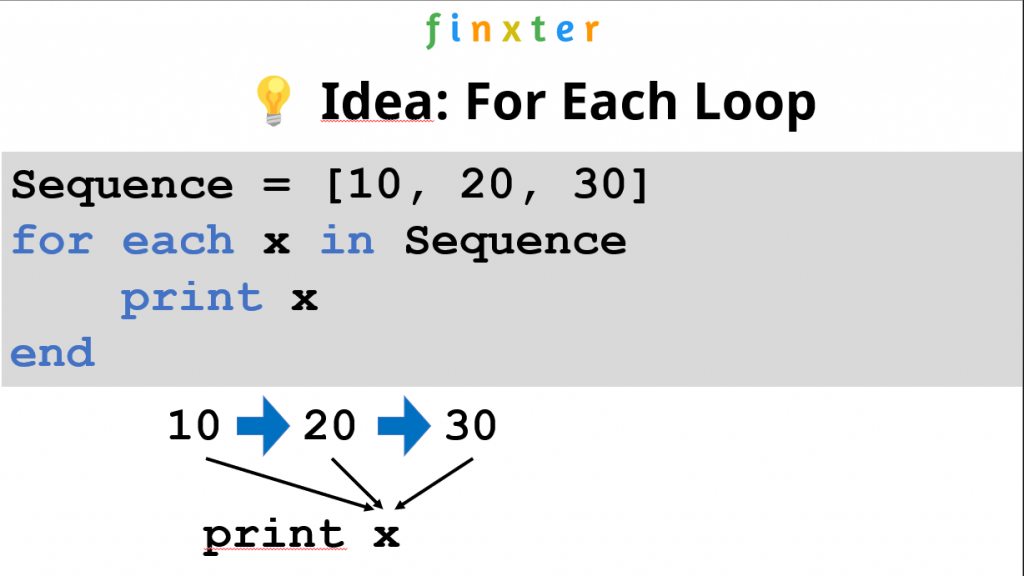# Python foreach Loop

💡 Question: Does Python have a `for each` or `foreach` loop? If so, how does it work? If not, what is the alternative?

This article will shed light on these questions. I’ll give you the summary first and dive into the details later:

Python has three alternatives to the “for each” loop:

1. A simple `for ... in ...` loop
2. A `map()` function
3. A list comprehension statement.

You’ll learn about those alternatives in the following paragraphs, so keep reading!

Let’s get started with the most important question:

## What is a “Foreach Loop”?

Definition: A `foreach` or `for each` loop is a programming control flow statement for iterating over elements in a sequence or collection. Unlike other loop constructs, the `foreach` loop iterates over all elements rather than maintaining a counter, loop variable, or checking a condition after each loop iteration.Figure: Example of a for each loop (pseudocode) that iterates over elements 10, 20, and 30 and prints their value.

Here are three examples of a `foreach` loop in three different programming languages PHP, C#, and Perl:

```// PHP
foreach (\$set as \$value) {
// Do something to \$value;
}

// C#
foreach (String val in array) {
console.writeline(val);
}

// Perl
foreach (1, 2, 3, 4) {
print \$_;
}```

## Does Python have a foreach Loop?

The Python language doesn’t support the keywords `foreach` or `for each` loops in a literal syntactical way. However, “for each” in Python is done using the “for … in …” expression. For example, to iterate over each element in the list `[10, 20, 30]` in Python, you’d write `for x in [10, 20, 30]`.

Here’s a full Python code example with semantical equivalence to a “foreach” statement:

```# 'foreach' or 'for each' in Python is done using 'for'
for x in [10, 20, 30]:
print(x)```

Output:

```10
20
30```

## “For Each” Meaning “Apply Function to Each Element”

If you’re reading this and you haven’t been satisfied with the answers provided so far, chances are that you’re really searching for the map function functionality in Python.

Many programming languages with “for each” support provide a syntax that applies a function to each element of an iterable like so:

````# Other programming languages:`
`foreach(function, iterable)````

This can be done in Python by means of the `map()` function:

``````# Python:
map(function, iterable)``````

Here’s a simple example of how you’d use the `map()` function in Python that applies the function `f` to each element of the list `[1, 2, 3]`, incrementing each of its elements by 1 to obtain `[2, 3, 4]`:

```lst = [1, 2, 3]

def f(x):
return x + 1

print(map(f, lst))
# [2, 3, 4]```

You can watch my explainer video on `map()` in the following video:

🌍 Learn More: Feel free to check out our full article on `map()` on the Finxter blog.

## “For Each” as Python List Comprehension

Python’s list comprehension feature is syntactical sugar to create a new iterable by applying a (possibly identity) function to each element of an existing iterable.

💡 Many coders would view the list comprehension feature as Python’s way to provide a functional “foreach” statement because it enables you to perform a function “for each” element of an iterable such as a sequence.

List comprehension is a compact way of creating lists. The simple formula is `[expression + context]`.

• Expression: What to do with each list element?
• Context: What elements to select? The context consists of an arbitrary number of `for` and `if` statements.

The example `[x+10 for x in [1, 2, 3]]` creates the list `[11, 12, 13]`.

```lst = [1, 2, 3]
new_lst = [x+10 for x in lst]
print(new_lst)
# [11, 12, 13]```

You can watch my explainer video on list comprehension in case you’re interested in how it works: